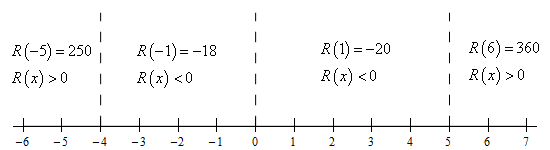Paul's Online Notes
Home / Calculus I / Review / Functions
Show Mobile Notice Show All Notes Hide All Notes
Mobile Notice
You appear to be on a device with a "narrow" screen width (i.e. you are probably on a mobile phone). Due to the nature of the mathematics on this site it is best views in landscape mode. If your device is not in landscape mode many of the equations will run off the side of your device (should be able to scroll to see them) and some of the menu items will be cut off due to the narrow screen width.

### Section 1.1 : Review : Functions

27. Find the domain of $$h\left( x \right) = \sqrt {{x^4} - {x^3} - 20{x^2}}$$.

Show All Steps Hide All Steps

Hint : We need to avoid negative numbers under the square root and because the quantity under the root is a polynomial we know that it can only change sign if it goes through zero and so we first need to determine where it is zero.
Start Solution

In this case we need to avoid square roots of negative numbers so we need to require,

${x^4} - {x^3} - 20{x^2} = {x^2}\left( {{x^2} - x - 20} \right) = {x^2}\left( {x - 5} \right)\left( {x + 4} \right) \ge 0$

Once we have the polynomial in factored form we can see that the left side will be zero at $$x = 0$$, $$x = - 4$$ and $$x = 5$$. Because the quantity under the radical is a polynomial we know that it can only change sign if it goes through zero and so these are the only points the only places where the polynomial on the left can change sign.

Hint : Because the polynomial can only change sign at these points we know that it will be the same sign in each region defined by these points and so all we need to know is the value of the polynomial as a single point in each region.
Show Step 2

Here is a number line giving the value/sign of the polynomial at a test point in each of the region defined by these three points. To make it a little easier to read the number line let’s define the polynomial under the radical to be,

$R\left( x \right) = {x^4} - {x^3} - 20{x^2} = {x^2}\left( {x - 5} \right)\left( {x + 4} \right)$

Now, here is the number line,Hint : Now all we need to do is write down the values of $$x$$ where the polynomial under the root will be positive or zero and we’ll have the domain. Be careful with the points where the polynomial is zero.
Show Step 3

The domain will then be all the points where the polynomial under the root is positive or zero and so the domain is,

${\rm{Domain : }} - \infty < x \le - 4,\,\,\,x = 0,\,\,\,5 \le x < \infty$

In this case we need to be very careful and not miss $$x = 0$$. This is the point separating two regions which give negative values of the polynomial, but it will give zero and so it also part of the domain. This point is often very is very easy to miss.